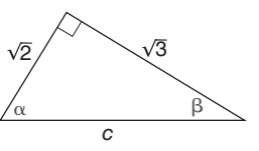Chapter 11.1, Problem 5E### Elementary Geometry for College St...

6th Edition
Daniel C. Alexander + 1 other
ISBN: 9781285195698

#### Solutions

Chapter
Section### Elementary Geometry for College St...

6th Edition
Daniel C. Alexander + 1 other
ISBN: 9781285195698
Textbook Problem
1 views

# In Exercises 1 to 6, find sin     α and sin     β for the triangle shown.To determine

To find:

The angle sinαandsinβ

Explanation

In a right angle triangle,

1) The side PQ, which is opposite to the right angle PRQ is called the hypotenuse.

(The hypotenuse is the longest side of the right triangle.)

2) The side RQ is called the adjacent side of angle θ.

3) The side PR is called the opposite side of angle θ.

Hence, the sine ratio for an acute angle is oppositehypotenuse

Given:

By using Pythagoras theorem, that is

c2=a2+b2

From, the diagram we have c=3andb=5

c2=(2)2+(3)2c2=2+3c=5

For angle α, the opposite side is 3 and hypotenuse side is 5

### Still sussing out bartleby?

Check out a sample textbook solution.

See a sample solution

#### The Solution to Your Study Problems

Bartleby provides explanations to thousands of textbook problems written by our experts, many with advanced degrees!

Get Started

#### Prove Property 3 of integrals.

Single Variable Calculus: Early Transcendentals, Volume I

#### let f(x) = x 1, g(x) = x+1, and h(x) = 2x3 1. Find the rule for each function. 17. f-hg

Applied Calculus for the Managerial, Life, and Social Sciences: A Brief Approach

#### 2. Define the terms population, sample, parameter and statistic.

Statistics for The Behavioral Sciences (MindTap Course List)

#### Simplify: 08

Elementary Technical Mathematics

#### The general solution to (for x, y > 0) is: a) y = ln x + C b) c) y = ln(ln x + C) d)

Study Guide for Stewart's Single Variable Calculus: Early Transcendentals, 8th

#### True or False: is a convergent series.

Study Guide for Stewart's Multivariable Calculus, 8th